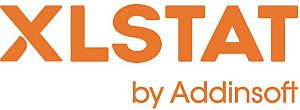# XLSTAT - Classification and Regression Trees

## Use of classification and regression trees

Classification and regression trees are methods that deliver models that meet both explanatory and predictive goals. Two of the strengths of this method are on the one hand the simple graphical representation by trees, and on the other hand the compact format of the natural language rules.

We distinguish the following two cases where these modeling techniques should be used:

• Use classification trees to explain and predict the belonging of objects (observations, individuals) to a class, on the basis of explanatory quantitative and qualitative variables.
• Use regression tree to build an explanatory and predicting model for a dependent quantitative variable based on explanatory quantitative and qualitative variables.

## Algorithms for classification and regression trees in XLSTAT

XLSTAT uses the CHAID, exhaustive CHAID, QUEST and C&RT (Classification and Regression Trees) algorithms.

Classification and regression trees apply to quantitative and qualitative dependent variables. In the case of a Discriminant analysis or logistic regression, only qualitative dependent variables can be used. In the case of a qualitative depending variable with only two categories, the user will be able to compare the performances of both methods by using ROC curves.

## Results for classification and regression trees in XLSTAT

Among the numerous results provided, XLSTAT can display the classification table (also called confusion matrix) used to calculate the percentage of well-classified observations. The proportion of well-classified positive events is called the sensitivity. The specificity is the proportion of well-classified negative events. If you vary the threshold probability from which an event is to be considered positive, the sensitivity and specificity will also vary.

When only two classes are present in the dependent variable, the ROC (Receiver Operating Characteristics) curve may also be displayed. It is the curve of points (1-specificity, sensitivity). It can be used for comparison with other models as it displays the performance of a model. The area under the curve (or AUC) is a synthetic index calculated for ROC curves. The AUC corresponds to the probability such that a positive event has a higher probability given to it by the model than a negative event. For an ideal model, AUC=1 and for a random model, AUC = 0.5. A model is usually considered good when the AUC value is greater than 0.7. A well-discriminating model must have an AUC of between 0.87 and 0.9. A model with an AUC greater than 0.9 is excellent.

## Validation for classification and regression trees

You are advised to validate the model on a validation sample wherever possible. XLSTAT has several options for generating a validation sample automatically.This analysis is available in the XLStat-Basic addin for Microsoft ExcelKovach Computing Services (KCS) was founded in 1993 by Dr. Warren Kovach. The company specializes in the development and marketing of inexpensive and easy-to-use statistical software for scientists, as well as in data analysis consulting.

### Get in Touch

• Email:
sales@kovcomp.com